## 前言

1. 如何求解线性空间的基？
2. 向量的子空间、零空间、列空间、行空间、左零空间都是什么东西？怎么求解？
3. 如何用线性代数的知识来拟合数据？
4. 机器学习、图像处理中常见的“特征向量”究竟是什么东西？它和变换矩阵有什么联系？

## 阶梯形矩阵

### 阶梯形矩阵

1. 若有零行（元素全为0的行），则零行应在最下方；
2. 非零首元（即非零行的第一个不为零的元素）的列标号随行标号的增加而严格递增。

$\begin{bmatrix} 2 & 0 & 2 & 1 \\ 0 & 5 & 2 & -2 \\ 0 & 0 & 3 & 2 \\ 0 & 0 & 0 & 0 \end{bmatrix}$

### 行简化阶梯形矩阵

1. 它是阶梯形矩阵；
2. 非零首元所在的列除了非零首元外，其余元素全为0。

$\begin{bmatrix} 2 & 0 & 2 & 1 \\ 0 & 5 & 2 & -2 \\ 0 & 0 & 3 & 2 \\ 0 & 0 & 0 & 0 \end{bmatrix}$

1. 它是行简化阶梯形矩阵；
2. 非零首元都为1

### 将矩阵化简成行最简阶梯形

$\begin{bmatrix} 1 & 2 & 1 & 1 & 7\\ 1 & 2 & 2 & -1 & 12\\ 2 & 4 & 0 & 6 & 4 \end{bmatrix}$

，使用初等变换可以将这个矩阵转换成如下的形式：

$\begin{bmatrix} 1 & 2 & 1 & 1 & 7\\ 1 & 2 & 2 & -1 & 12\\ 2 & 4 & 0 & 6 & 4 \end{bmatrix} \rightarrow \begin{bmatrix} 1 & 2 & 1 & 1 & 7\\ 0 & 0 & 1 & -2 & 5\\ 2 & 4 & 0 & 6 & 4 \end{bmatrix} \rightarrow \begin{bmatrix} 1 & 2 & 1 & 1 & 7\\ 0 & 0 & 1 & -2 & 5\\ 0 & 0 & -2 & 4 & -10 \end{bmatrix} \rightarrow \begin{bmatrix} 1 & 2 & 1 & 1 & 7\\ 0 & 0 & 1 & -2 & 5\\ 0 & 0 & 0 & 0 & 0 \end{bmatrix} \rightarrow \begin{bmatrix} 1 & 2 & 0 & 3 & 2\\ 0 & 0 & 1 & -2 & 5\\ 0 & 0 & 0 & 0 & 0 \end{bmatrix}$

$\left\{ \begin{eqnarray} x_1 + 2x_2 + x_3 + x_4 &=& 7 \\\ x_1 + 2x_2 + 2x_3 - x_4 &=& 12 \\\ 2x_1 + 4x_2 + 6x_4 &=& 4 \end{eqnarray} \right.$

$\begin{bmatrix} 1 & 2 & 1 & 1 & 7\\ 1 & 2 & 2 & -1 & 12\\ 2 & 4 & 0 & 6 & 4 \end{bmatrix}$

$\begin{bmatrix} 1 & 2 & 0 & 3 & 2\\ 0 & 0 & 1 & -2 & 5\\ 0 & 0 & 0 & 0 & 0 \end{bmatrix}$

$\left\{ \begin{eqnarray} x_1 &=& 2 - 2x_2 - 3x_4 \\\ x_3 &=& 5 + 2x_4 \end{eqnarray} \right.$

$\begin{bmatrix} x_{ 1 } \\ x_{ 2 } \\ x_{ 3 } \\ x_{ 4 } \end{bmatrix}=\begin{bmatrix} 2 \\ 0 \\ 5 \\ 0 \end{bmatrix}+x_{ 2 }\underbrace { \begin{bmatrix} -2 \\ 1 \\ 0 \\ 0 \end{bmatrix} }_{ \vec{\mathbf{a}} } +x_{ 4 }\underbrace{\begin{bmatrix} -3 \\ 0 \\ 2 \\ 1 \end{bmatrix}}_{\vec{\mathbf{b}}}$

## 线性子空间

1. 零向量 0 在 $\mathbf{W}$ 中。
2. 加法封闭：如果 $\vec{\mathbf{u}}$ 和 $\vec{\mathbf{v}}$ 是 $\mathbf{W}$ 的元素，则向量和 $\mathbf{\vec{\mathbf{u}}+\vec{\mathbf{v}}}$ 是 $\mathbf{W}$ 的元素。
3. 标量乘法封闭：如果 $\vec{\mathbf{u}}$ 是 $\mathbf{W}$ 的元素而 $c$ 是标量，则标量积 $c\vec{\mathbf{u}}$ 是 $\mathbf{W}$ 的元素。

### 零空间

$\begin{bmatrix} 1 & 1 & 1 & 1 \\ 1 & 2 & 3 & 4 \\ 4 & 3 & 2 & 1 \end{bmatrix} \begin{bmatrix} x_1 \\ x_2 \\ x_3 \\ x_4 \end{bmatrix} = \begin{bmatrix} 0 \\ 0 \\ 0 \end{bmatrix}$

$\left\{ \begin{eqnarray} x_1 + x_2 + x_3 + x_4 &=& 0 \\\ x_1 + 2x_2 + 3x_3 + 4x_4 &=& 0 \\\ 4x_1 + 3x_2 + 2x_4 + x_4 &=& 0 \end{eqnarray} \right.$

$\begin{bmatrix} 1 & 1 & 1 & 1 & 0 \\ 1 & 2 & 3 & 4 & 0 \\ 4 & 3 & 2 & 1 & 0 \end{bmatrix}$

$\begin{bmatrix} 1 & 0 & -1 & -2 & 0 \\ 0 & 1 & 2 & 3 & 0 \\ 0 & 0 & 0 & 0 & 0 \end{bmatrix}$

$\begin{bmatrix} x_{ 1 } \\ x_{ 2 } \\ x_{ 3 } \\ x_{ 4 } \end{bmatrix}=x_{ 3 }\underbrace { \begin{bmatrix} 1 \\ -2 \\ 1 \\ 0 \end{bmatrix} }_{ \vec{\mathbf{a}} } +x_{ 4 }\underbrace{\begin{bmatrix} 2 \\ -3 \\ 0 \\ 1 \end{bmatrix}}_{\vec{\mathbf{b}}}$

$N(\mathbf{A}) = span\left(\begin{bmatrix} 1 \\ -2 \\ 1 \\ 0 \end{bmatrix} \begin{bmatrix} 2 \\ -3 \\ 0 \\ 1 \end{bmatrix}\right)$

### 列空间

$C(\mathbf{A}) = span(\vec{v_1}, \vec{v_2}, \ldots, \vec{v_n})$

$\begin{bmatrix} 1 & 0 & -1 & -2 \\ 0 & 1 & 2 & 3 \\ 0 & 0 & 0 & 0 \end{bmatrix}$

import numpy as np
a = np.matrix('1 1 1 1;1 2 3 4; 0 0 1 0')
print a.ndim  # 2（维度）
print np.rank(a)  # 2（a.ndim 的别名，已经过时）
print np.linalg.matrix_rank(a)  # 3（秩）


### 左零空间

$N(\mathbf{A}^T) = \left\{ \vec{\mathbf{x}} | \mathbf{A}^{T} \vec{\mathbf{x}} = \vec{\mathbf{0}} \right\} = \left\{ \vec{\mathbf{x}} | \vec{\mathbf{x}}^{T} \mathbf{A} = \vec{\mathbf{0}}^{T} \right\}$

## 最小二乘逼近

$\underset{n\times k}{\mathbf{A}}\vec{\mathbf{x}} = \vec{\mathbf{b}}$

$\vec{a_1}\vec{\mathbf{x}} + \vec{a_2}\vec{\mathbf{x}} + \ldots + \vec{a_k}\vec{\mathbf{x}} = \vec{\mathbf{b}}$

$\left\| \begin{bmatrix} \vec{b_1}-\vec{v_1}\\ \vec{b_2}-\vec{v_2}\\ \ldots\\ \vec{b_n}-\vec{v_n}\\ \end{bmatrix} \right\|^2 = (b_1-v_1)^2 + (b_2-v_2)^2 + \ldots + (b_n-v_n)^2$

$\mathbf{A}\vec{\mathbf{x}^*} = Proj_{C(\mathbf{A})}\vec{\mathbf{b}}$

$\mathbf{A}^T\mathbf{A}\vec{\mathbf{x}^*} = \mathbf{A}^T\vec{\mathbf{b}}$

### 实例1：求解方程

$\left\{ \begin{eqnarray} x + y &=& 3 \\\ x - y &=& -2 \\\ y &=& 1 \end{eqnarray} \right.$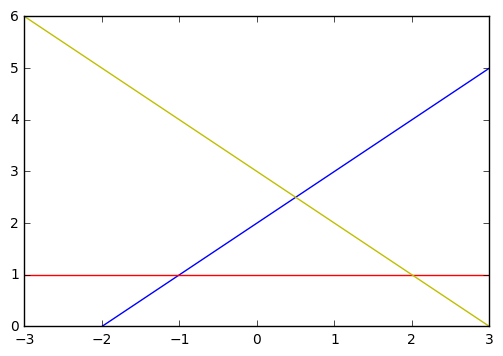$\underbrace{ \begin{bmatrix} 1 & 1 \\ 1 & -1 \\ 0 & 1 \end{bmatrix} }_{\mathbf{A}} \underbrace{ \begin{bmatrix} x \\ y \end{bmatrix} }_{\vec{\mathbf{x}}} = \underbrace{ \begin{bmatrix} 3 \\ -2 \\ 1 \end{bmatrix} }_{\vec{\mathbf{b}}}$

\begin{align} \begin{bmatrix} 1 & 1 & 0 \\ 1 & -1 & 1\\ \end{bmatrix} \begin{bmatrix} 1 & 1 \\ 1 & -1 \\ 0 & 1 \end{bmatrix} \begin{bmatrix} x^* \\ y^* \end{bmatrix} & = \begin{bmatrix} 1 & 1 & 0 \\ 1 & -1 & 1\\ \end{bmatrix} \begin{bmatrix} 3 \\ -2 \\ 1 \end{bmatrix} \\\ \begin{bmatrix} 2 & 0 \\ 0 & 3 \end{bmatrix} \begin{bmatrix} x^* \\ y^* \end{bmatrix} & = \begin{bmatrix} 1 \\ 6 \end{bmatrix} \end{align}

$\begin{bmatrix} x^* \\ y^* \end{bmatrix} = \begin{bmatrix}\frac{1}{2} & 0 \\ 0 & \frac{1}{3}\end{bmatrix} \begin{bmatrix} 1 \\ 6 \end{bmatrix} = \begin{bmatrix} \frac{1}{2} \\ 2 \end{bmatrix}$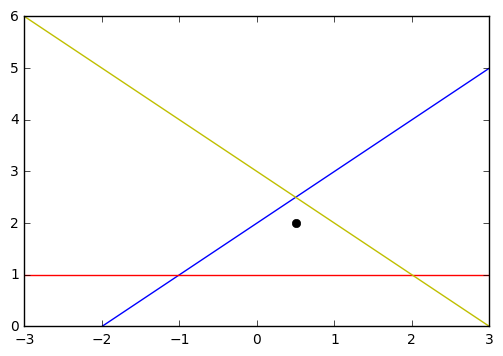numpy.linalg.lstsq 的返回包括四个部分：

1. 最小二乘逼近解。如果 b 是二维的，那么这个逼近的结果有多个列，每一列是一个逼近解。对于上例，逼近解就是 $\begin{bmatrix}0.5 \\ 2 \end{bmatrix}$ 。
2. 残差。即每一个 b - a*x 的长度的和。对于上例，残差是 1.5 。
3. 矩阵 a 的秩。对于上例，矩阵 a 的秩为 2 。
4. 矩阵 a 的奇异值。对于上例，矩阵 a 的奇异值为 $\begin{bmatrix}1.73205081 \\ 1.41421356\end{bmatrix}$

### 实例2：线性回归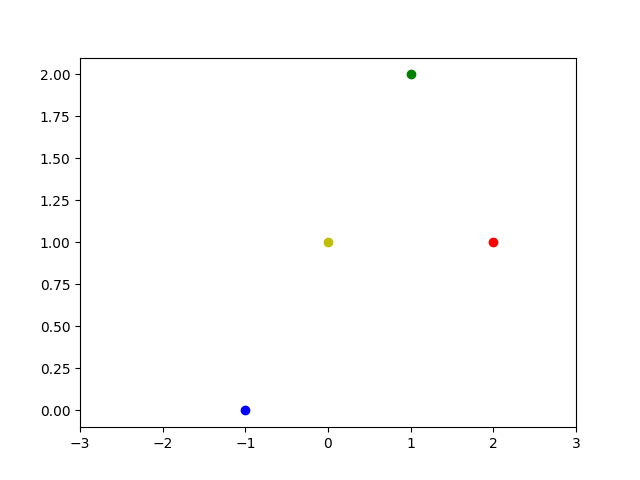$\left\{ \begin{eqnarray} f(-1) &= -m + b = 0\\\ f(0) &= 0 + b = 1\\\ f(1) &= m + b = 2\\\ f(2) &= 2m + b = 1 \end{eqnarray} \right.$

$\underbrace{ \begin{bmatrix} -1 & 1 \\ 0 & 1 \\ 1 & 1 \\ 2 & 1 \end{bmatrix} }_{\mathbf{A}} \underbrace{ \begin{bmatrix} m\\ b \end{bmatrix} }_{\vec{\mathbf{x}}} = \underbrace{ \begin{bmatrix} 0\\ 1\\ 2\\ 1 \end{bmatrix} }_{\vec{\mathbf{b}}}$

\begin{align} \begin{bmatrix} -1 & 0 & 1 & 2 \\ 1 & 1 & 1 & 1 \end{bmatrix} \begin{bmatrix} -1 & 1 \\ 0 & 1 \\ 1 & 1 \\ 2 & 1 \end{bmatrix} \begin{bmatrix} m^*\\ b^* \end{bmatrix} &= \begin{bmatrix} -1 & 0 & 1 & 2 \\ 1 & 1 & 1 & 1 \end{bmatrix} \begin{bmatrix} 0\\ 1\\ 2\\ 1 \end{bmatrix}\\\ \begin{bmatrix} 6 & 2 \\ 2 & 4 \end{bmatrix} \begin{bmatrix} m^*\\ b^* \end{bmatrix} &= \begin{bmatrix} 4\\ 4 \end{bmatrix} \end{align}

$\begin{bmatrix}m^*\\b^*\end{bmatrix} = \frac{1}{20}\begin{bmatrix}4 & -2\\-2 & 6\end{bmatrix}\begin{bmatrix}4 \\ 4\end{bmatrix} = \frac{1}{20}\begin{bmatrix}8 \\ 16\end{bmatrix} = \begin{bmatrix}\frac{2}{5} \\ \frac{4}{5}\end{bmatrix}$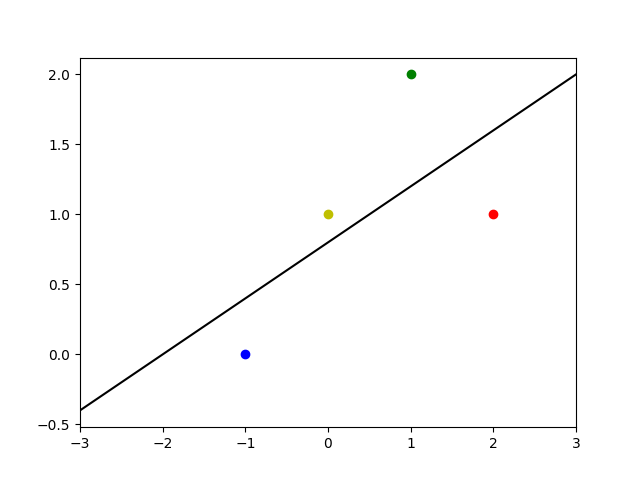Python 示例如下：

## 特征向量

“特征”在模式识别和图像处理中是非常常见的一个词汇。我们要认识和描绘一件事物，首先得找出这个事物的特征。同样的道理，要让计算机识别一件事物，首先就要让计算机学会理解或者抽象出事物的特征。什么样的东西能当成特征呢？那必须是能“放之四海皆准”的依据，不论个体如何变换，都能从中找到这类群体共有的特点。例如，计算机视觉中常用的 SIFT 特征点 是一种很经典的用于视觉跟踪的特征点，即使被跟踪的物体的尺度、角度发生了变化，这种特征点依然能够找到关联。在机器学习中， 特征向量选取也是整个机器学习系统中非常重要的一步。

$\mathbf{T}(\vec{\mathbf{v}}) = \underbrace{\mathbf{A}}_{n\times n}\vec{\mathbf{v}} = \underbrace{\lambda}_{特征值} \overbrace{\vec{\mathbf{v}}}^{特征向量}$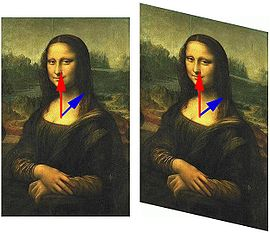### 求解特征值

eq: 1 »

$det(\lambda \mathbf{I}_n - \underbrace{\mathbf{A}}_{n\times n}) = 0$

\begin{align} det\left( \lambda \begin{bmatrix} 1 & 0 \\ 0 & 1 \end{bmatrix}-\begin{bmatrix} 1 & 2 \\ 4 & 3 \end{bmatrix} \right) &=0 \\ det\left( \begin{bmatrix} \lambda & 0 \\ 0 & \lambda \end{bmatrix}-\begin{bmatrix} 1 & 2 \\ 4 & 3 \end{bmatrix} \right) &=0 \\ det\left( \begin{bmatrix} \lambda -1 & -2 \\ -4 & \lambda -3 \end{bmatrix} \right) &=0 \end{align}

\begin{align} (\lambda -1)(\lambda -3)-8 & =0 \\ \lambda ^{ 2 }-4\lambda -5 &=0 \\ (\lambda - 5)(\lambda +1) &= 0\end{align}

### 求解特征向量

eq: 2 »

$\mathbf{E}_{\lambda}=N(\lambda I_n - \mathbf{A})$

${ E }_{ \lambda }=N\left( \lambda I_{ n }-\begin{bmatrix} 1 & 2 \\ 4 & 3 \end{bmatrix} \right) =N\left( \lambda \begin{bmatrix} 1 & 0 \\ 0 & 1 \end{bmatrix}-\begin{bmatrix} 1 & 2 \\ 4 & 3 \end{bmatrix} \right) =N\left( \begin{bmatrix} \lambda -1 & -2 \\ -4 & \lambda -3 \end{bmatrix} \right)$

${ E }_{ 5 }=N\left( \begin{bmatrix} 4 & -2 \\ -4 & 2 \end{bmatrix} \right)$

${ E }_{ 5 }= span\left(\begin{bmatrix}\frac{1}{2} \\ 1 \end{bmatrix}\right)$

${ E }_{ -1 }= span\left(\begin{bmatrix}1 \\ -1 \end{bmatrix}\right)$